# What Is An Inverse Function? (12 Common Questions Answered)

Inverse functions help us to “undo” the action of a function.  When composed, a function and its inverse leave an input unchanged (the identity function).

So, what is an inverse function?  An inverse function f-1(x) is the “reverse” of a function f(x). The x and y variables (and thus their domain and range) are flipped, and their composition gives us the identify f(f-1(x)) = x = f-1(f(x)). A function must be bijective (injective & surjective, or one to one & onto) to have an inverse.

Of course, some functions are their own inverses.  Functions can also intersect their inverses at certain specific points on the line y = x.

Let’s get started.

## What Is An Inverse Function?

An inverse function f-1(x) is the “opposite” or “reverse” of another function f(x).  Their x and y variables (and thus domain and range) are flipped, and the composition of these two functions gives us the identify

• f(f-1(x)) = x = f-1(f(x))

### When Does A Function Have An Inverse Function?

A function f(x) has an inverse function f-1(x) when f(x) is a bijection.  A function is a bijection when it is both surjective (onto) and injective (one to one).

#### Surjective Functions

A function f(x) is surjective if, for every y in the range, there is an x in the domain such that f(x) = y (that is, for each y value in the domain, we can find an x value that maps to y).

A function that is surjective has a right inverse g(x) such that f(g(x)) = x, but g(x) is not an inverse unless f(x) is also injective.

#### Injective Functions

A function f(x) is injective if f(x1) = f(x2) implies that x1 = x2 (that is, the function is one to one, meaning it passes the horizontal line test).

A function that is injective has a left inverse g(x) such that g(f(x)) = x, but g(x) is not an inverse unless f(x) is also surjective.

### How To Find An Inverse Function

To find an inverse function for f(x):

• First, rewrite f(x) as y [remember that y = f(x) for the purposes of a function].
• Next, switch the x and y variables.
• Then, solve for x.
• Finally, y is the inverse function g(x)  [y = g(x)]

Let’s look at some examples to see how to find the inverse of a function.

#### Example 1: Finding The Inverse Of A Function

Consider the function f(x) = 2x + 6.

This function is bijective, so it has an inverse function g(x).

• f(x) = 2x + 6
• y = 2x + 6  [replace f(x) with y]
• x = 2y + 6  [switch x and y]
• x – 6 = 2y
• (x – 6) / 2 = y  [solve for y]
• (x – 6) / 2 = g(x)  [replace y with g(x)]

So, the inverse function of f(x) = 2x + 6 is g(x) = (x – 6) / 2.  You can see the graphs of both functions below.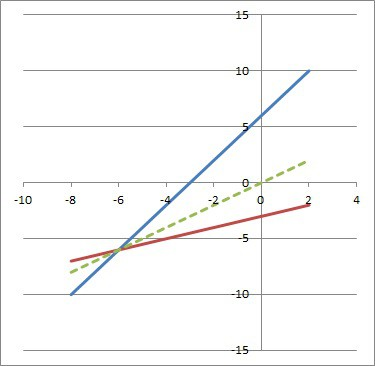The function f(x) = 2x + 6 (blue line) and its inverse g(x) = (x – 6) / 2 (red line). Note that the inverse g(x) is the graph of f(x) reflected across the line y = x (green dashed line).

#### Example 2: Finding The Inverse Of A Function

Consider the function f(x) = x3 + 3x2 + 3x + 1.

This function is bijective, so it has an inverse function g(x).

• f(x) = x3 + 3x2 + 3x + 1
• f(x) = (x + 1)3  [factor by recognizing binomial coefficients from Pascal’s Triangle]
• y = (x + 1)3  [replace f(x) with y]
• x = (y + 1)3  [switch x and y]
• x1/3 = y + 1
• x1/3 – 1 = y  [solve for y]
• x1/3 – 1 = g(x)  [replace y with g(x)]

So, the inverse function of f(x) = x3 + 3x2 + 3x + 1 is g(x) = x1/3 – 1.  You can see the graphs of both functions below.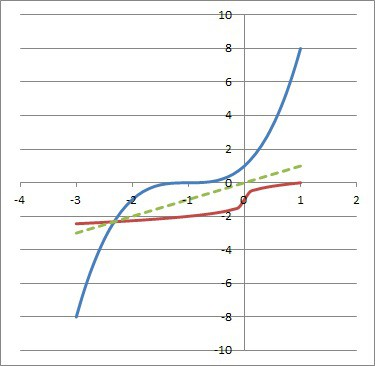The function f(x) = x3 + 3x2 + 3x + 1 (blue curve) and its inverse g(x) = x1/3 – 1 (red curve). Note that the inverse g(x) is the graph of f(x) reflected across the line y = x (green dashed line).

### How To Graph An Inverse Function

To graph an inverse function of a bijective function f(x), we simply switch x and y, as we did above.  From a graphical standpoint, this means that we simply reflect the graph of f(x) across the line y = x.

One simple way to do this is to take a point (a, b) on the graph of f(x) and plot the point (b, a) on the graph of g(x).  That is, switch the x and y coordinates of a point on the graph of f(x) to find a corresponding point on the graph of g(x).

Let’s take a look at an example to see how it works.

#### Example: Graphing An Inverse Function

Consider the function f(x) = x3.

This function is bijective, so it has an inverse function g(x).

We can see the graph of f(x) = x3 below.

We can see that some of the points on the graph of f(x) are:

• (-2, -8)
• (-1, -1)
• (0, 0)
• (1, 1)
• (2, 8)

To find corresponding points on the graph of g(x), all we need to do is switch the x and y coordinates of the points from the graph of f(x).  We can see this in the following table:

Now we can use these points to sketch a graph of g(x), which we can see (along with the graph of f(x)) below: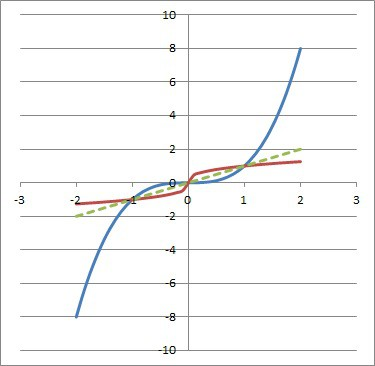The function f(x) = x3 (blue curve) and its inverse g(x) = x1/3 (red curve). Note that the inverse g(x) is the graph of f(x) reflected across the line y = x (green dashed line).

Note that the graph of g(x) is the graph of f(x) reflected across the line y = x (this line is the identity function, where every input returns an output of itself).

### Can An Inverse Function Be The Same?

An inverse function can be the same as the function itself.  In this case, we say that f(x) is self-inverse.

A self-inverse function satisfies the following equations:

• f(x) = f-1­(x)  [the function is its own inverse]
• f(f(x)) = x  [this follows from the first equation, since f(f-1­(x)) = x and f(x) = f-1­(x)]

Some examples of functions that are self-inverse include:

• f(x) = x  [the identity function]
• f(x) = -x  [the negative of the identity function]
• f(x) = a – x  [for any real value of a]
• f(x) = 1 / x  [the reciprocal function]
• f(x) = a / x  [for any real value of a]

Note that the f(x) = a / x class of functions excludes the value x = 0 (due to the zero denominator).

### Can An Inverse Function Be Negative?

An inverse function can be negative for some of its output values.  In fact, an inverse function must be negative for some output values if f(x) is a bijective function with a range of all real numbers.

For example, the function f(x) = x3 is bijective and has a range of all real numbers.  It has the inverse function g(x) = x1/3.

For any value of x that is negative, the inverse function g(x) will also be negative (since the real cube root of a negative number is negative).  For instance, g(-8) = (-8)1/3 = -2.

Some inverse functions can have a negative sign, such as f(x) = -x (which is its own inverse).

### Can An Even Function Have An Inverse Function?

An even function f(x) defined on the domain of real numbers cannot have an inverse function.  The reason is that an even function is not injective (one to one), since it fails the horizontal line test.  A function that is not injective is not bijective, and so it cannot have an inverse function.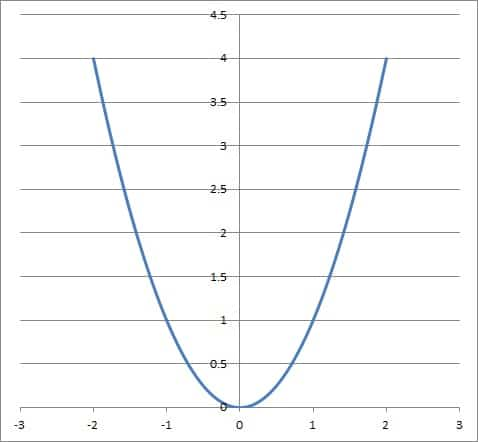The function f(x) = x2 (blue curve) is not injective (one to one) when defined on the domain of all real numbers, so it does not have an inverse.

Remember that if f(x) is even, then f(-x) = f(x), meaning that the points (a, b) and (-a, b) are both on the graph of f(x).  Since both of these points are on the horizontal line y = b, the graph fails the horizontal line test, meaning f(x) is not one-to-one.

However, if we restrict the domain of an even function to the set of nonnegative real numbers, then it may be one-to-one and may have an inverse function.

For example, consider the function f(x) = x2 on the domain and range of nonnegative real numbers.  The function is bijective, so it has an inverse: g(x) = √x.

Note that the inverse g(x) is also restricted to the domain and range of nonnegative real numbers.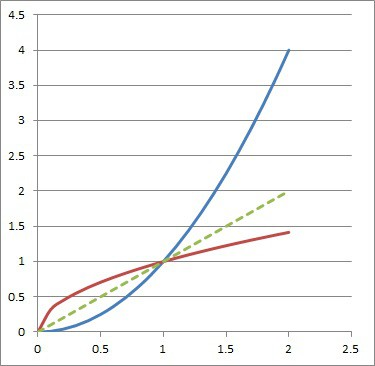The function f(x) = x2 (blue curve) and its inverse g(x) = x1/2 (red curve). Note that the inverse g(x) is the graph of f(x) reflected across the line y = x (green dashed line).

### Can Inverse Functions Be Parallel?

Inverse functions can be parallel in some cases.  Consider the linear function f(x) = mx + b for nonzero m.

Since f(x) is injective, it has an inverse, which we will find now:

• f(x) = mx + b
• y = mx + b
• x = my + b
• x – b = my
• (x – b) / m = y
• (x – b) / m = g(x)

So, the inverse of the linear function f(x) = mx + b is the linear function g(x) = (x – b) / m.  The two lines will be parallel if they have the same slope, but different y intercepts.

Equating the slopes, we get:

• slope of f(x) = slope of g(x)
• m = 1 / m
• m2 = 1
• m = +1 or m = -1

In the first case (m = +1), the y-intercept of f(x) is b, and the y-intercept of g(x) is –b / m = -b / 1 = -b.  So, f(x) and g(x) are parallel in this case.

In the second case (m = -1), the y-intercept of f(x) is b, and the y-intercept of g(x) is –b / m = -b / -1 = b.  So, f(x) and g(x) are the same line in this case (that is, they are coincident, not parallel).

So, any line of the form f(x) = x + b (for a real number b that is nonzero) is parallel to its inverse function g(x) = x – b.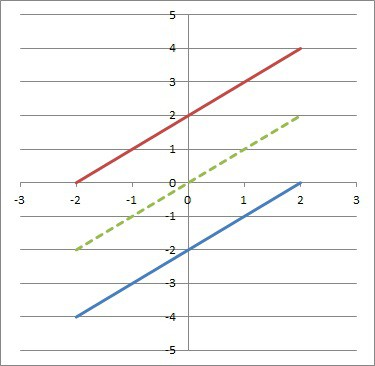The function f(x) = x – 2 (blue line) and its inverse g(x) = x + 2 (red curve). Note that the inverse g(x) is the graph of f(x) reflected across the line y = x (green dashed line).

### Can Inverse Functions Intersect?

Inverse functions can intersect.  However, this will only occur at points on the line y = x (the identity function).

This is because if a point (a, b) is on the graph of the function f(x), then the point (b, a) is on the graph of g(x).

In order to have f(x) = g(x) at a point (a, b), we must have (a, b) = (b, a).  This means a = b, and so the point has the form (a, a), which lies on the line y = x.The function f(x) = x3 (blue curve) and its inverse g(x) = x1/3 (red curve) intersect at the points (-1, -1), (0, 0), and (1, 1), which all lie on the line y = x (dashed green line).

### Do Inverse Functions Have The Same Domain?

Inverse functions do not always have the same domain.  In fact, they “trade” domains and ranges.

For example, if the function f(x) has a domain of D and a range of R, then its inverse function g(x) has a domain of R and a range of D.

#### Example 1: A Function With The Same Domain As Its Inverse

Consider the function f(x) = x, with a domain of the real numbers and a range of the real numbers.

Its inverse function g(x) = x has a domain of the real numbers and a range of the real numbers.

So, the function f(x) and its inverse function g(x) have the same domain in this case.

#### Example 2: A Function With A Different Domain Than Its Inverse

Consider the function f(x) = 2x, with a range of {1, 3, 5} and a range of {2, 6, 10}.

Then the inverse function g(x) = x / 2 has a domain of {2, 6, 10} and a range of {1, 3, 5}.

In this case, the domain of the inverse {2, 6, 10} is not the same as the domain of the function {1, 3, 5}.

### Is Inverse Function The Same As Reciprocal?

An inverse function is not the same as the reciprocal function (also know as the multiplicative inverse).  However, the notation can be confusing.

For a function f(x), its inverse is often denoted by f-1(x).  However, in this context, the “-1” superscript does not mean the reciprocal of f(x).

If we mean the reciprocal of f(x), we should write [f(x)]-1 or 1 / f(x).

### Is Inverse Function The Same As Derivative?

An inverse function is not the same as the derivative.  For a function f(x):

• The inverse g(x) of f(x) is the function that satisfies f(g(x)) = x = g(f(x)).
• The derivative f’(x) of f(x) is the function that tells us the slope of f(x) at any value of x.

## Conclusion

Now you know what inverse functions are and the answers to some common questions about them.